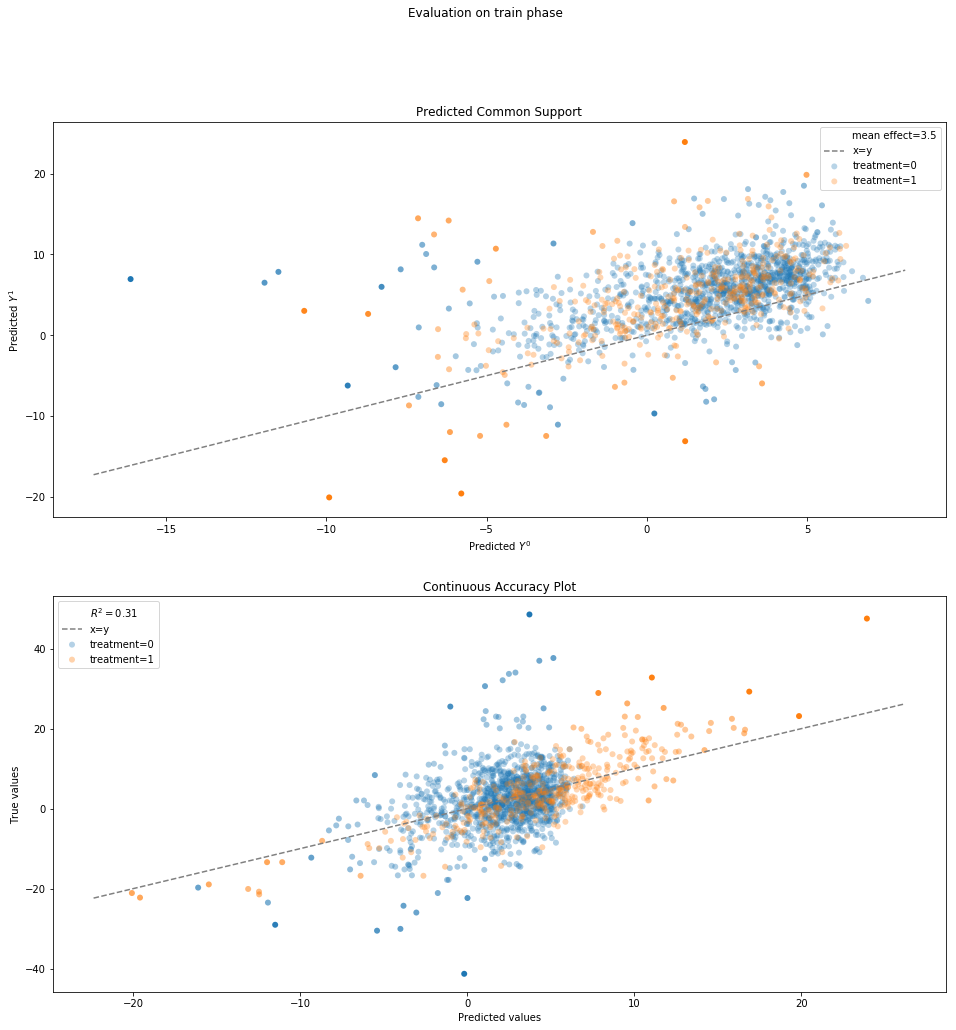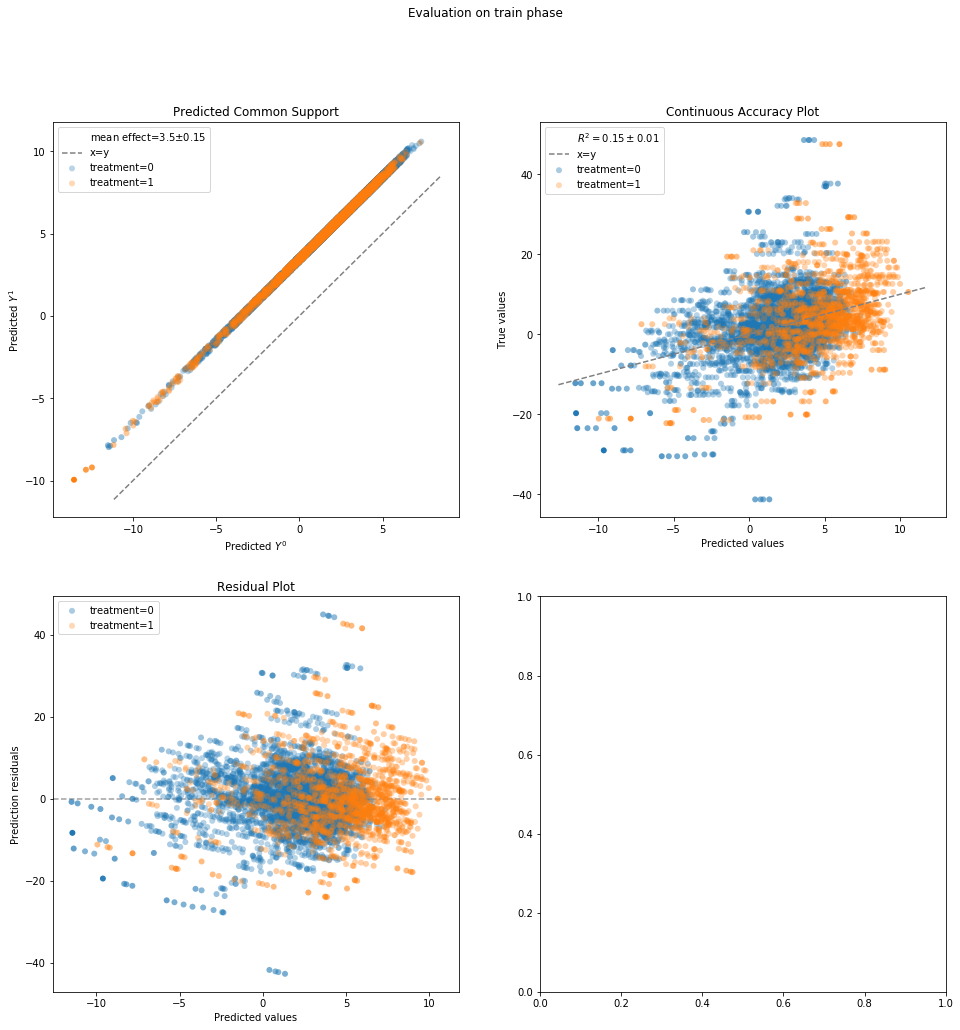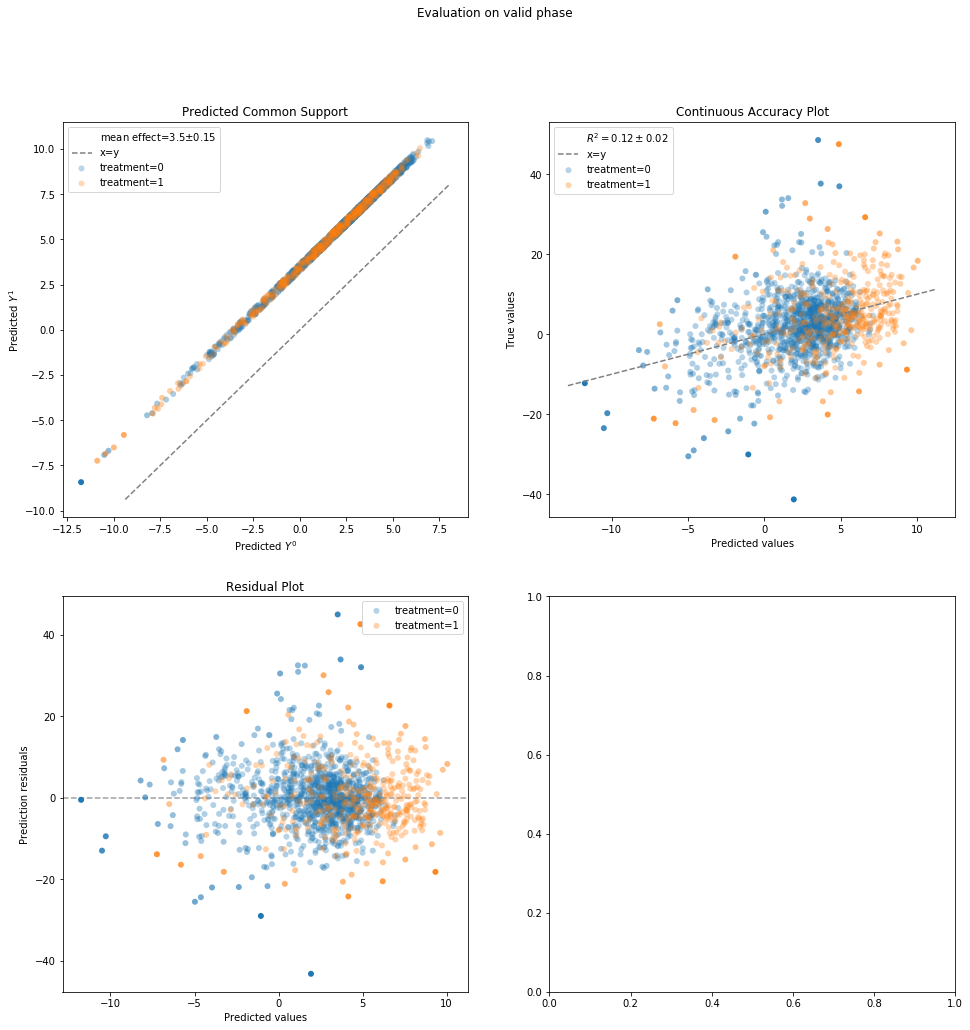# Direct Outcome Prediction Model¶

Also known as standardization

In :
%matplotlib inline
from sklearn.linear_model import LinearRegression

from causallib.estimation import Standardization, StratifiedStandardization
from causallib.evaluation import OutcomeEvaluator


#### Data:¶

The effect of smoking cessation on weight loss.
Data example is taken from Hernan and Robins Causal Inference Book

In :
data = load_nhefs()

Out:
age race sex smokeintensity smokeyrs wt71 active_1 active_2 education_2 education_3 education_4 education_5 exercise_1 exercise_2 age^2 wt71^2 smokeintensity^2 smokeyrs^2 qsmk wt82_71
0 42 1 0 30 29 79.04 0 0 0 0 0 0 0 1 1764 6247.3216 900 841 0 -10.093960
1 36 0 0 20 24 58.63 0 0 1 0 0 0 0 0 1296 3437.4769 400 576 0 2.604970
2 56 1 1 20 26 56.81 0 0 1 0 0 0 0 1 3136 3227.3761 400 676 0 9.414486
3 68 1 0 3 53 59.42 1 0 0 0 0 0 0 1 4624 3530.7364 9 2809 0 4.990117
4 40 0 0 20 19 87.09 1 0 1 0 0 0 1 0 1600 7584.6681 400 361 0 4.989251

## "Standard" Standardization (S-Learner)¶

A single model (the S in S-Learner) is trained with the treatment assignment as an additional feature.
During inference, the model assigns a single treatment value for all samples, thus predicting the potential outcome of all samples.

In :
std = Standardization(LinearRegression())
std.fit(data.X, data.a, data.y)

Out:
Standardization(encode_treatment=False, predict_proba=False,
learner=LinearRegression(copy_X=True, fit_intercept=True, n_jobs=None, normalize=False))
##### Outcome Prediction¶

The model can be used to predict individual outcomes:
The potential outcome under each intervention (columns correspond to treatment values)

In :
ind_outcomes = std.estimate_individual_outcome(data.X, data.a)

Out:
qsmk 0 1
0 4.271756 7.734378
1 6.337239 9.799861
2 1.989807 5.452429
3 -4.261624 -0.799002
4 2.290883 5.753505

The model can be used to predict population outcomes,
By aggregating the individual outcome prediction (e.g., mean or median).
Providing agg_func which is defaulted to 'mean'

In :
median_pop_outcomes = std.estimate_population_outcome(data.X, data.a, agg_func="median")
median_pop_outcomes.rename("median", inplace=True)

mean_pop_outcomes = std.estimate_population_outcome(data.X, data.a, agg_func="mean")
mean_pop_outcomes.rename("mean", inplace=True)

pop_outcomes = mean_pop_outcomes.to_frame().join(median_pop_outcomes)
pop_outcomes

Out:
mean median
qsmk
0 1.747216 2.391416
1 5.209838 5.854038
##### Effect Estimation¶

Similarly, Effect estimation can be done on either individual or population level, depending on the outcomes provided.

Population level effect using population outcomes:

In :
std.estimate_effect(mean_pop_outcomes, mean_pop_outcomes)

Out:
diff    3.462622
dtype: float64

Population level effect using individual outcome, but asking for aggregation (default behaviour):

In :
std.estimate_effect(ind_outcomes, ind_outcomes, agg="population")

Out:
diff    3.462622
dtype: float64

Individual level effect using inidividual outcomes:
Since we're using a binary treatment with linear regression on a standard model,
the difference is same for all individuals, and is equal to the coefficient of the treatment varaible.
This is usually refered to as homogenuous effect.

In :
print(std.learner.coef_)

3.4626218292258635

Out:
effect_type diff
0 3.462622
1 3.462622
2 3.462622
3 3.462622
4 3.462622

Multiple types of effect are also supported:

In :
std.estimate_effect(ind_outcomes, ind_outcomes,

Out:
effect_type diff ratio
0 3.462622 1.810585
1 3.462622 1.546393
2 3.462622 2.740179
3 3.462622 0.187488
4 3.462622 2.511479

### Treament one-hot encoded¶

For multi-treatment cases, where treatments are coded as 0, 1, 2, ... but have no ordinal interpretation,
It is possible to make the model encode the treatment assignment vector as one hot matrix.

In :
std = Standardization(LinearRegression(), encode_treatment=True)
std.fit(data.X, data.a, data.y)
pop_outcomes = std.estimate_population_outcome(data.X, data.a, agg_func="mean")
std.estimate_effect(mean_pop_outcomes, mean_pop_outcomes)

Out:
diff    3.462622
dtype: float64

## Stratified Standarziation (T-Learner)¶

While standardization above can be viewed as a "complete pooled" estimator,
as it includes both treatment groups together,
Stratified Standardization can viewed as "complete unpooled" one,
as it completly stratifies the dataset by treatment values and learns a different model for each treatment group.

In :
std = StratifiedStandardization(LinearRegression())
std.fit(data.X, data.a, data.y)

Out:
StratifiedStandardization(learner=LinearRegression(copy_X=True, fit_intercept=True, n_jobs=None, normalize=False))

Checking the core learner we can see that it actually has two models (the T in T-Learner), indexed by the treatment value:

In :
std.learner

Out:
{0: LinearRegression(copy_X=True, fit_intercept=True, n_jobs=None, normalize=False),
1: LinearRegression(copy_X=True, fit_intercept=True, n_jobs=None, normalize=False)}

We can apply same analysis as above.

In :
pop_outcomes = std.estimate_population_outcome(data.X, data.a, agg_func="mean")
std.estimate_effect(mean_pop_outcomes, mean_pop_outcomes)

Out:
diff    3.462622
dtype: float64

While the average population-level effect is the same, the individual effect is now heterogenuous.
However, this flexibility in modelling does not come for free, since each model now uses less data and therefore has less statistical power.

In :
ind_outcomes = std.estimate_individual_outcome(data.X, data.a)

Out:
effect_type diff
0 3.389345
1 4.774150
2 7.653848
3 0.754123
4 6.769839

We can see that internally, when asking for some potential outcome,
the model simply applies the model trained on the group of that treatment:

In :
potential_outcome = std.estimate_individual_outcome(data.X, data.a)
direct_prediction =  std.learner.predict(data.X)
(potential_outcome == direct_prediction).all()

Out:
True

#### Providing complex scheme of learners¶

When supplying a single learner to the standardization above, the model simply duplicates it for each treatment value.
However, it is possible to specify a different model for each treatment value explicitly.
For example, in cases where the treated are more complex than the untreated
(because, say, background of those choosed to be treated),
it is possible to specify them with a more expressive model:

In :
learner = {0: LinearRegression(),
std = StratifiedStandardization(learner)
std.fit(data.X, data.a, data.y)
std.learner

Out:
{0: LinearRegression(copy_X=True, fit_intercept=True, n_jobs=None, normalize=False),
learning_rate=0.1, loss='ls', max_depth=3,
max_features=None, max_leaf_nodes=None,
min_impurity_decrease=0.0, min_impurity_split=None,
min_samples_leaf=1, min_samples_split=2,
min_weight_fraction_leaf=0.0, n_estimators=100,
n_iter_no_change=None, presort='auto',
random_state=None, subsample=1.0, tol=0.0001,
validation_fraction=0.1, verbose=0, warm_start=False)}
In :
ind_outcomes = std.estimate_individual_outcome(data.X, data.a)

Out:
qsmk 0 1
0 4.174723 1.611459
1 5.715355 10.336035
2 1.069715 8.876902
3 -3.004029 -2.302499
4 1.303085 13.128340
In :
std.estimate_effect(ind_outcomes, ind_outcomes)

Out:
diff    3.457361
dtype: float64

## Evaluation¶

#### Simple evaluation¶

In :
plots = ["common_support", "continuous_accuracy"]
evaluator = OutcomeEvaluator(std)
evaluator._regression_metrics.pop("msle")  # We have negative values and this is log transforms
results = evaluator.evaluate_simple(data.X, data.a, data.y, plots=plots)Results show the results for each treatment group separetly and also combined:

In :
results.scores

Out:
expvar mae mse mdae r2
model_strata
0 0.134184 4.984365 48.001726 3.892460 0.134184
1 0.620207 4.069050 28.994209 3.366938 0.620207
actual 0.305271 4.748815 43.110264 3.725702 0.305271

#### Thorough evaluation¶

In :
plots=["common_support", "continuous_accuracy", "residuals"]
evaluator = OutcomeEvaluator(Standardization(LinearRegression()))
results = evaluator.evaluate_cv(data.X, data.a, data.y,
plots=plots)In :
results.scores

Out:
expvar mae mse mdae r2
phase fold model_strata
train 0 0 0.120261 4.836409 45.095388 3.746441 0.120261
1 0.132930 5.901975 60.400336 4.410732 0.132930
actual 0.144336 5.110460 49.031645 3.904165 0.144336
1 0 0.131070 5.107275 51.199296 3.884197 0.131070
1 0.144062 6.151565 68.033377 4.652069 0.144062
actual 0.151529 5.375854 55.528828 4.108958 0.151529
2 0 0.120794 5.090933 50.587440 3.886812 0.120794
1 0.148452 6.134991 68.006193 4.638904 0.148452
actual 0.144978 5.359452 55.067343 4.071345 0.144978
3 0 0.131716 5.022344 49.429310 3.887024 0.131716
1 0.141006 6.177875 66.850313 4.631242 0.141006
actual 0.150930 5.319981 53.916538 4.110475 0.150930
4 0 0.140784 4.945217 45.100058 3.908426 0.140784
1 0.136182 6.021523 64.623919 4.768222 0.136182
actual 0.158225 5.222447 50.128931 4.092505 0.158225
valid 0 0 0.111470 5.647694 64.084827 4.335749 0.107987
1 0.142307 6.890068 87.642987 5.358262 0.141837
actual 0.131870 5.968179 70.161932 4.677565 0.129600
1 0 0.073147 4.676988 38.546913 3.813148 0.072158
1 0.106360 5.967635 57.060633 4.766350 0.106358
actual 0.107703 5.009925 43.322745 4.104752 0.107220
2 0 0.142695 4.714920 40.273362 3.827672 0.136704
1 0.077705 5.901309 57.512428 4.737501 0.077290
actual 0.150331 5.020963 44.720382 4.030534 0.146741
3 0 0.096127 5.004752 44.597650 3.872913 0.094266
1 0.102488 5.774340 62.998246 4.299598 0.098466
actual 0.120879 5.202083 49.315751 3.940780 0.118549
4 0 0.045712 5.362004 63.960017 3.855272 0.043081
1 0.169576 6.190794 68.560090 4.350113 0.168172
actual 0.093212 5.574514 65.139523 3.865244 0.092509
In :
results.models

Out:
[Standardization(encode_treatment=False, predict_proba=False,
learner=LinearRegression(copy_X=True, fit_intercept=True, n_jobs=None, normalize=False)),
Standardization(encode_treatment=False, predict_proba=False,
learner=LinearRegression(copy_X=True, fit_intercept=True, n_jobs=None, normalize=False)),
Standardization(encode_treatment=False, predict_proba=False,
learner=LinearRegression(copy_X=True, fit_intercept=True, n_jobs=None, normalize=False)),
Standardization(encode_treatment=False, predict_proba=False,
learner=LinearRegression(copy_X=True, fit_intercept=True, n_jobs=None, normalize=False)),
Standardization(encode_treatment=False, predict_proba=False,
learner=LinearRegression(copy_X=True, fit_intercept=True, n_jobs=None, normalize=False))]
In [ ]: